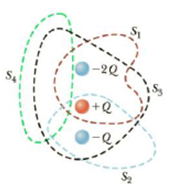Chapter 15, Problem 47P

Chapter
Section
Textbook Problem

Four closed surfaces, S1 through S4, together with the charges −2Q, Q, and −Q. are sketched in Figure P15.47. (The colored lines are the intersections of the surfaces with the page.) Find the electric flux through each surface.Figure P15.47

To determine
The electric flux through each surface.

Explanation

Formula to calculate the electric flux is,

ϕE=qinsideε0

• qinside is the charge inside the surface.
• ε0 is the permittivity of free space.

The charge inside S1 is,

qinside=Q2Q=Q

Therefore,

ϕE=Qε0

Formula to calculate the electric flux is,

ϕE=qinsideε0

• qinside is the charge inside the surface.
• ε0 is the permittivity of free space.

The charge inside S2 is,

qinside=QQ=0

Therefore,

ϕE=0

Formula to calculate the electric flux is,

ϕE=qinsideε0

• qinside is the charge inside the surface

Still sussing out bartleby?

Check out a sample textbook solution.

See a sample solution

The Solution to Your Study Problems

Bartleby provides explanations to thousands of textbook problems written by our experts, many with advanced degrees!

Get Started